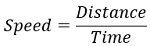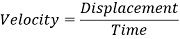# Difference Between Speed and Velocity

Both speed and velocity are the terms used in reference to the motion of the object. However, the crucial difference between speed and velocity is that speed is concerned with the motion of the object with time irrespective of the direction of motion. As against, velocity is associated with the motion of the object with time in a specific direction only.

Whenever in any discussion, we talk about the motion of a body or object, then terms like distance, displacement, and direction is taken into account in references to the quantities like speed and velocity. The terms speed and velocity are sometimes interchangeably used by people but these two terms hold a different meaning. Thus, its interchangeable usage is quite incorrect.

Here in this article, we will see how speed and velocity are differentiated.

## Content: Speed Vs Velocity

### Comparison Chart

Basis for ComparisonSpeedVelocity
Quantity TypeScalarVector
Given ass = distance/timev = displacement/time
MagnitudeOnly positiveCan be either positive, negative or zero.
Expressed asx km/hy km/h with direction
Effect of change of directionWith the change in direction, no change is considered on the magnitude of speed.Change in direction puts effect on the velocity thus change in direction is not advisable in case of velocity.
Graphical representationThe area under graph is the overall distance covered.The area under graph is the overall displacement of the object.

### Definition of Speed

Speed is defined as the rate with which the object is covering a specific distance in unit time.

Suppose 2 bodies started the motion to cover a specific distance. One body moves fast than the other body and reaches the opposite end in a specific time duration. However, the other body takes more time to cover the same distance. This signifies that both the bodies are covering the same distance but at different time instants. This makes the difference between the speed of the two bodies.

The body reaching the destination in a lesser amount of time than the other possesses more speed. It can be said in another way, that the body with less speed travels less distance in the same time while the body with high speed travels more distance in that particular time.

Thus, speed is given as:The rate of motion with time is speed. The speed of the moving body has nothing to do with the direction of motion. So, only the magnitude is required to specify the speed of the body. In a general sense, speed is not a constant term as the object generally undergoes non-uniform motion thus rate with which the object is moving corresponds to its average speed.

### Definition of Velocity

Velocity is defined as the rate with which the moving body possesses overall displacement with respect to time. Its relation to speed is defined as the speed-oriented in a specific direction.

Suppose two bodies started moving at the same time instant from a specific position. After reaching a specific point towards the south direction, one of the bodies comes back to the initial position moving in the north direction. While the other body proceeds further in the south direction.

So, when the displacement of both the bodies is considered then concerning the same amount of time for both the motion, the overall displacement of the first body will be 0 because of the same starting and terminating points. While the overall displacement of the second body is some positive value.

Since,So, the velocity of the first body is 0 according to the magnitude and direction of motion. But the velocity of the second body will definitely be a positive value as it displaces in the same direction traveling the desired distance with respect to time. This is the reason; velocity is direction-dependent along with distance during motion. The velocity of the body can be uniform or variable in nature as it can be varied with variation in either speed, direction, or both simultaneously.

## Key Differences Between Speed and Velocity

1. The key factor of differentiation between speed and velocity is that speed is a scalar quantity thus only magnitude is associated with it. On the contrary, velocity is a vector quantity hence magnitude and direction both are taken into consideration.
2. Speed is given as the rate with which the body is moving to reach a specific distance with respect to time. While velocity is the rate of change of position of the body showcasing the overall displacement of the body with respect to time.
3. Speed is always measured as a positive value in terms of magnitude as it has nothing to do with direction so the ratio with distance and time provides a positive magnitude. However, the velocity of a moving body can be positive, negative, or zero as the displacement signifies the position of the body and in the case of the same initial and final point, the velocity of the moving body will be 0.
4. When the direction of a moving body is changed then the speed count goes the same as earlier because the change in direction does not significantly change the distance and time ratio. While as velocity specifies the change in position of the body with respect to time thus direction is crucially associated with velocity.
5. As the direction is a crucial parameter for defining speed and velocity thus speed is simply expressed as x km/hr, but velocity is y km/hr with direction (e.g. south).
6. In the speed-time graph, the region within the graph corresponds to the distance covered by the object at a certain time. However, in the velocity-time graph, the region within the graph corresponds to the overall displacement of the body in unit time.

### Conclusion

So, from this discussion, we can conclude that speed and distance are the two different quantities concerned with the motion of bodies with respect to time. Thus, it is said that speed is the distance-time graph while velocity is the gradient of displacement time graph.